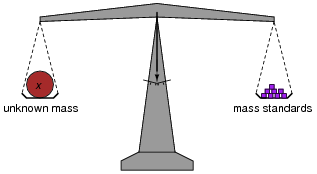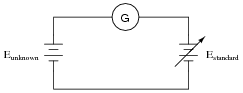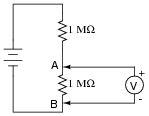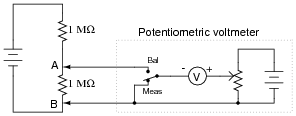# DC Metrology

## DC Electric Circuits

• #### Question 1

Define the following metrological terms:

• Uncertainty
• Accuracy
• Error
• Precision
• Traceability
• #### Question 2

In chemistry laboratories, balance scales are used to precisely measure the mass of various substances. How, exactly, is a laboratory “balance” scale used? What component of the scale primarily determines its accuracy?• #### Question 3

Explain how this electrical system functions in a similar manner to a laboratory “balance” scale:The circle with the letter “G” in it is a symbol for a galvanometer. What practical purpose might a system like this serve?

• #### Question 4

If a voltmeter is to be used to directly measure the voltage of an unknown source, it must first be calibrated so as to ensure an accurate measurement:What is the minimum number of points along the meter’s range that it needs to be calibrated at, given the assumption of perfectly linear response?

If a voltmeter is to be used to measure the voltage of an unknown source, as a differential indicator only, what is the minimum number of points along its range that it needs to be calibrated at?• #### Question 5

Explain the simplest way to perform a single-point calibration on a highly sensitive, precision voltmeter. How do you ensure the voltmeter is receiving a fixed input of known quantity, especially without having expensive calibration equipment available?

• #### Question 6

This voltage divider should output half the battery’s voltage between points A and B:However, if you perform this same experiment using a real voltmeter, the measurement obtained with the meter will be substantially different from what should be there, based on a prediction of 1/2Ebattery. Explain why the voltmeter registers as it does. What is it about this circuit that causes the measurement to be so far off from the prediction, when we know full well that other voltage divider circuits we’ve constructed do not exhibit any significant error?

• #### Question 7

The following voltmeter circuit avoids problems of “loading” when measuring high-resistance voltage sources. Describe how to operate this circuit, and how loading error is eliminated by using such a potentiometric instrument:• #### Question 8

General metrology used to be based upon standard artifacts, but the modern scientific trend is toward intrinsic standards. Explain what these two terms mean, as well as their significance in scientific work.

• #### Question 9

What technology is currently regarded as state-of-the-art for reproducing the standard volt?

• #### Question 10

What technology is currently regarded as state-of-the-art for reproducing the standard ohm?